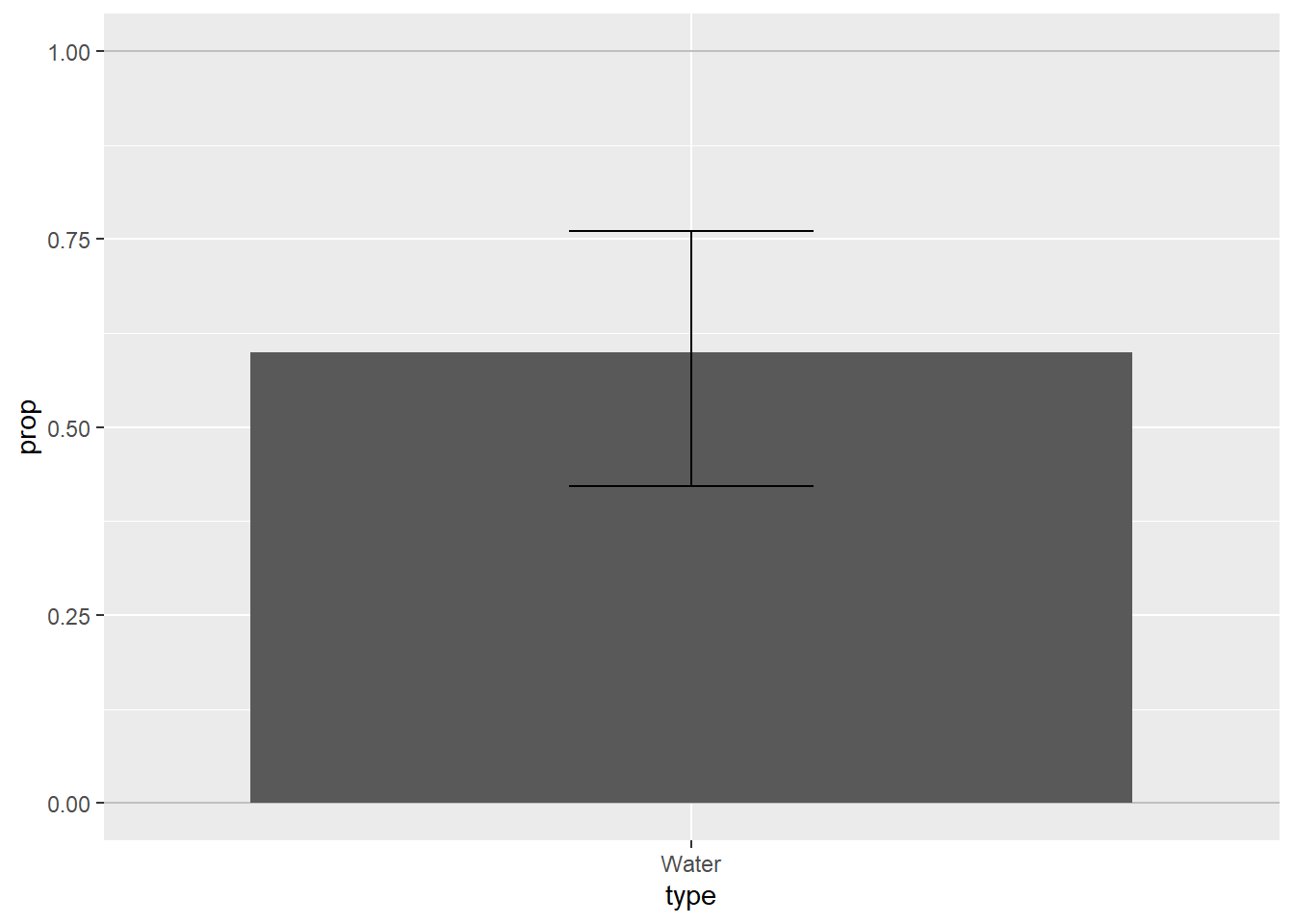Include your answers in this document in the sections below the rubric.

# Rubric

Answer the questions with the data example.

# Sample the Globe example

How can we estimate the proportion of water on the globe using a beach ball?

1. (2 p) What is a good sampling strategy to pick points at random from a sphere?

2. (2 p) How can this strategy be used to estimate the proportion of the globe covered by water?

3. (1 p) Enter the data we collected in class and compute the confidence interval. Let $$n=$$ total number of observations and let $$x=$$ the number of “successes” (number of water observations, land is a “failure”). Enter these numbers into the prop.test() and binom.test() functions below.

## notes for prop.test() and binom.test()
# x = number of "successes"
# n = total sample size

x = 21
n = 21 + 14

dat.globe <- data.frame(type = c("Water", "Land"), freq = c(x, n - x), prop = c(x, n - x) / n)
dat.globe
##    type freq prop
## 1 Water   21  0.6
## 2  Land   14  0.4
# prop.test() is an asymptotic (approximate) test for a binomial random variable
p.summary <- prop.test(x = x, n = n, conf.level = 0.95)
p.summary
##
##  1-sample proportions test with continuity correction
##
## data:  x out of n, null probability 0.5
## X-squared = 1.0286, df = 1, p-value = 0.3105
## alternative hypothesis: true p is not equal to 0.5
## 95 percent confidence interval:
##  0.4220904 0.7564794
## sample estimates:
##   p
## 0.6
# binom.test() is an exact test for a binomial random variable
b.summary <- binom.test(x = x, n = n, conf.level = 0.95)
b.summary
##
##  Exact binomial test
##
## data:  x and n
## number of successes = 21, number of trials = 35, p-value = 0.3105
## alternative hypothesis: true probability of success is not equal to 0.5
## 95 percent confidence interval:
##  0.4211177 0.7612919
## sample estimates:
## probability of success
##                    0.6
1. (2 p) Interpret the confidence interval for the proportion of water.

2. (3 p) Here’s a gimme! Label the plot: the title, $$x$$-, and $$y$$-axis.

Note how to add error bars using geom_errorbar(). First determine the CI bounds from the binom.test() previously, then set those as limits.

# get names of objects in b.summary
names(b.summary)
##  "statistic"   "parameter"   "p.value"     "conf.int"    "estimate"
##  "null.value"  "alternative" "method"      "data.name"
# here's the confidence interval bounds (the attribute tells us this is a 95% interval)
b.summary$conf.int ##  0.4211177 0.7612919 ## attr(,"conf.level") ##  0.95 b.summary$conf.int
##  0.4211177
b.summary$conf.int ##  0.7612919 library(ggplot2) p <- ggplot(data = subset(dat.globe, type == "Water"), aes(x = type, y = prop)) p <- p + geom_hline(yintercept = c(0, 1), alpha = 1/4) p <- p + geom_bar(stat = "identity") p <- p + geom_errorbar(aes(min = b.summary$conf.int, max = b.summary\$conf.int), width=0.25)
p <- p + scale_y_continuous(limits = c(0, 1))
print(p)